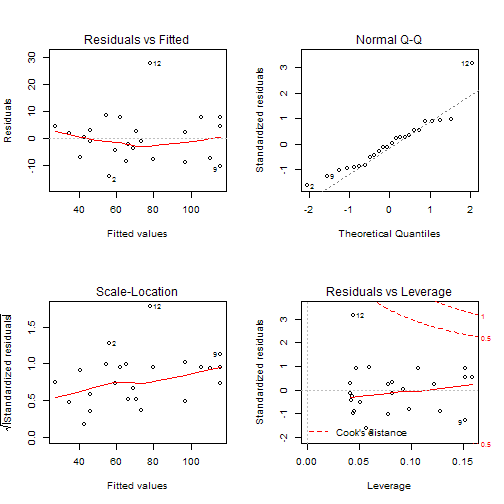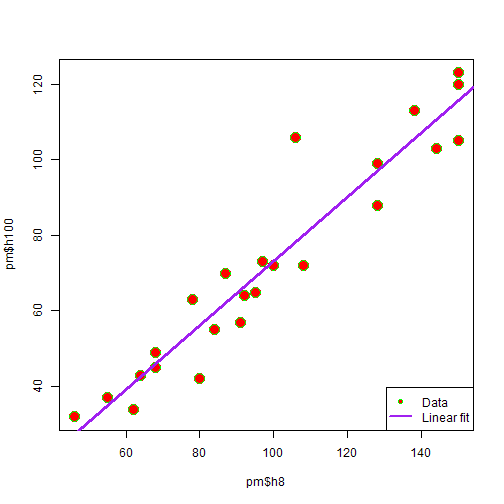``````pm <- read.csv(file = "c:\\R\\data\\dapengde_DummyR_PM25.csv")
m <- lm(pm\$h100 ~ pm\$h8)  # 线性拟合
m  # 查看模型，显示斜率和截距。
``````
``````##
## Call:
## lm(formula = pm\$h100 ~ pm\$h8)
##
## Coefficients:
## (Intercept)        pm\$h8
##     -11.471        0.846
``````
``````summary(m)  # 模型的详细总结报告。
``````
``````##
## Call:
## lm(formula = pm\$h100 ~ pm\$h8)
##
## Residuals:
##     Min      1Q  Median      3Q     Max
## -14.247  -7.113  -0.397   4.508  27.744
##
## Coefficients:
##             Estimate Std. Error t value Pr(>|t|)
## (Intercept)  -11.471      6.013   -1.91     0.07 .
## pm\$h8          0.847      0.058   14.58  8.6e-13 ***
## ---
## Signif. codes:  0 '***' 0.001 '**' 0.01 '*' 0.05 '.' 0.1 ' ' 1
##
## Residual standard error: 8.94 on 22 degrees of freedom
## Multiple R-squared: 0.906,   Adjusted R-squared: 0.902
## F-statistic:  213 on 1 and 22 DF,  p-value: 8.64e-13
``````
``````# 下面对拟合结果作图。
par(mfrow = c(2, 2))  # 把一张作图纸上分成两行两列。par() 在作图时很有用，请查看帮助。
plot(m)
````````````# 作图完毕。 给散点图添加拟合直线。
par(mfrow = c(1, 1))
plot(pm\$h8, pm\$h100, cex = 2, pch = 21, bg = "red", col = "green")
abline(m, col = "purple", lwd = 3)  # 添加趋势线
legend("bottomright", pch = c(21, NA), lty = c(NA, 1), legend = c("Data", "Linear fit"),
pt.bg = "red", col = c("green", "purple"), lwd = c(NA, 2))
``````## 有用的信息：

### 8 Responses to “R 菜鸟入门篇 第04篇 模型”

1. R是个好软件，n年前修一门课要求用，之后就荒废了。这个月特别忙，路过一下留脚印，以后有时间仔细读读你的R之菜鸟入门。

• 你应该已经不是菜鸟了，不用在我写的这个上面浪费时间了。不如去读 The R Book。

2. 飘过来学习一下，赞一下你这个系列~~

• 牛人来了啊，欢迎欢迎。今天才读完你翻译的25招，只悔自己孤陋寡闻，既然你都写过了，我怀疑这个系列还没有继续写下去的必要了。

3. R的数据真丰富啊，有些看不明白，比如那四个图里面的第四个是啥意思呢？

• 我也不太懂，好像是衡量野点的影响的。

4. dapeng再懂点统计学就好了，揉着一块儿讲，多有用啊。

• 这个光荣的任务还是交给小语妈吧。